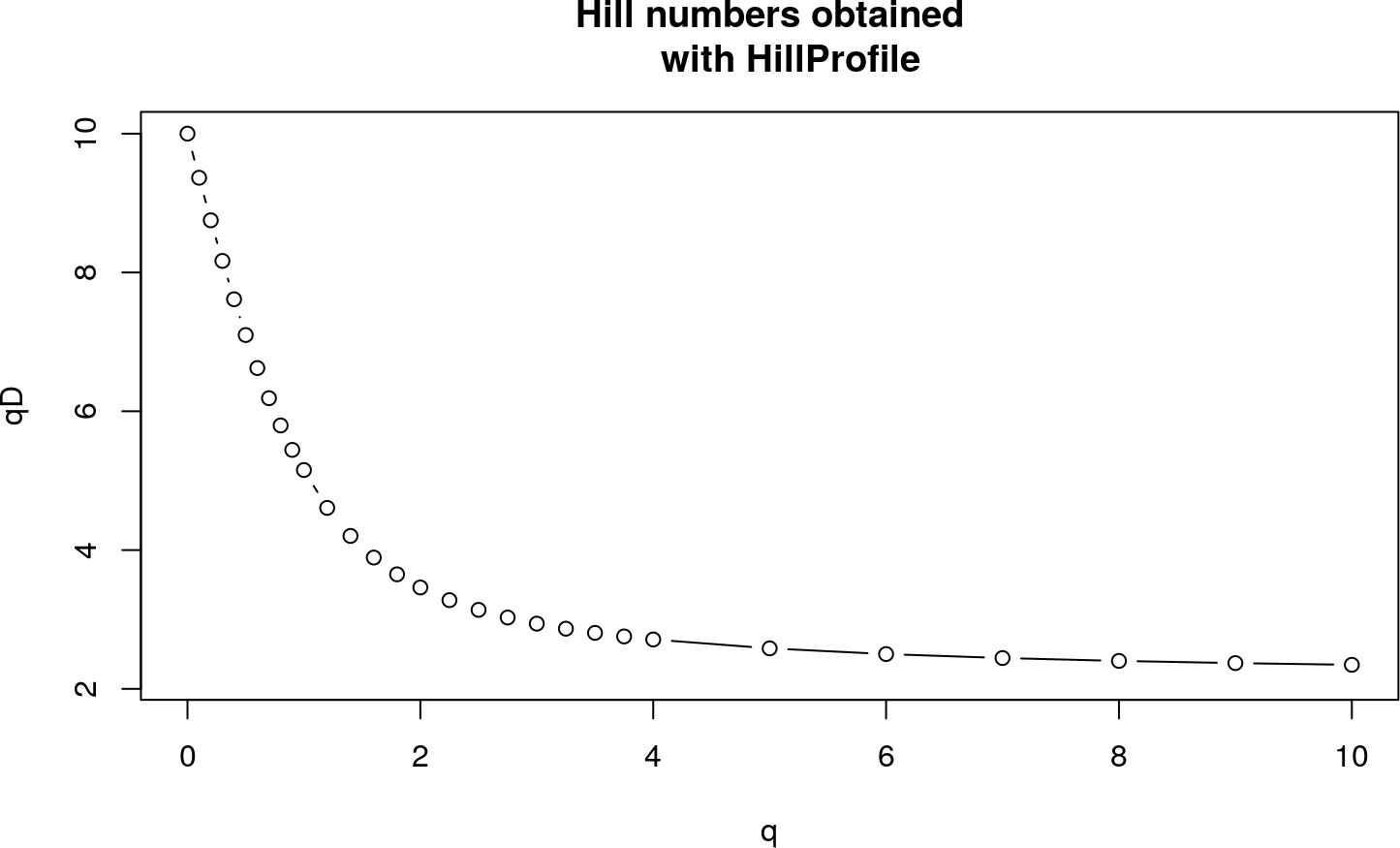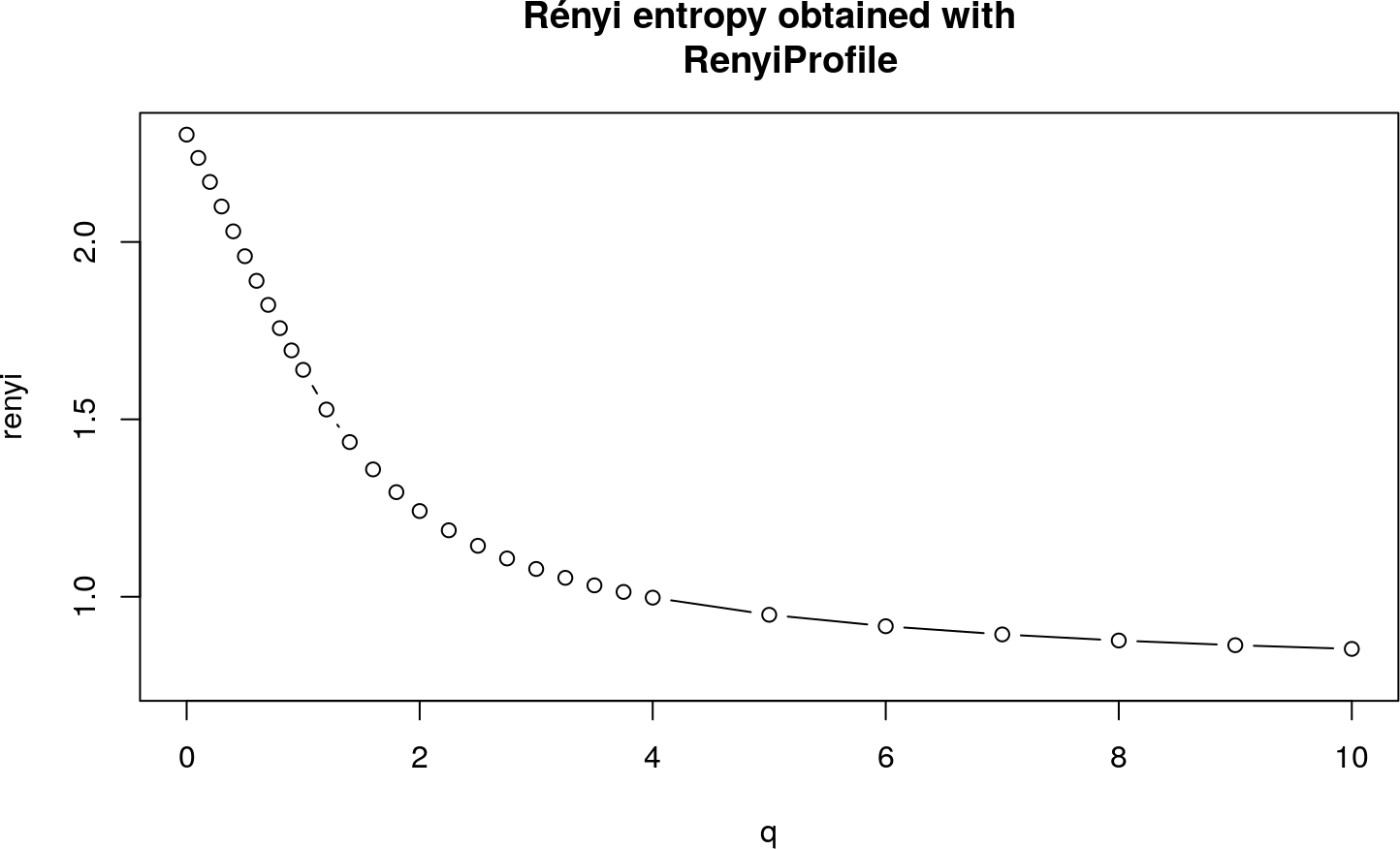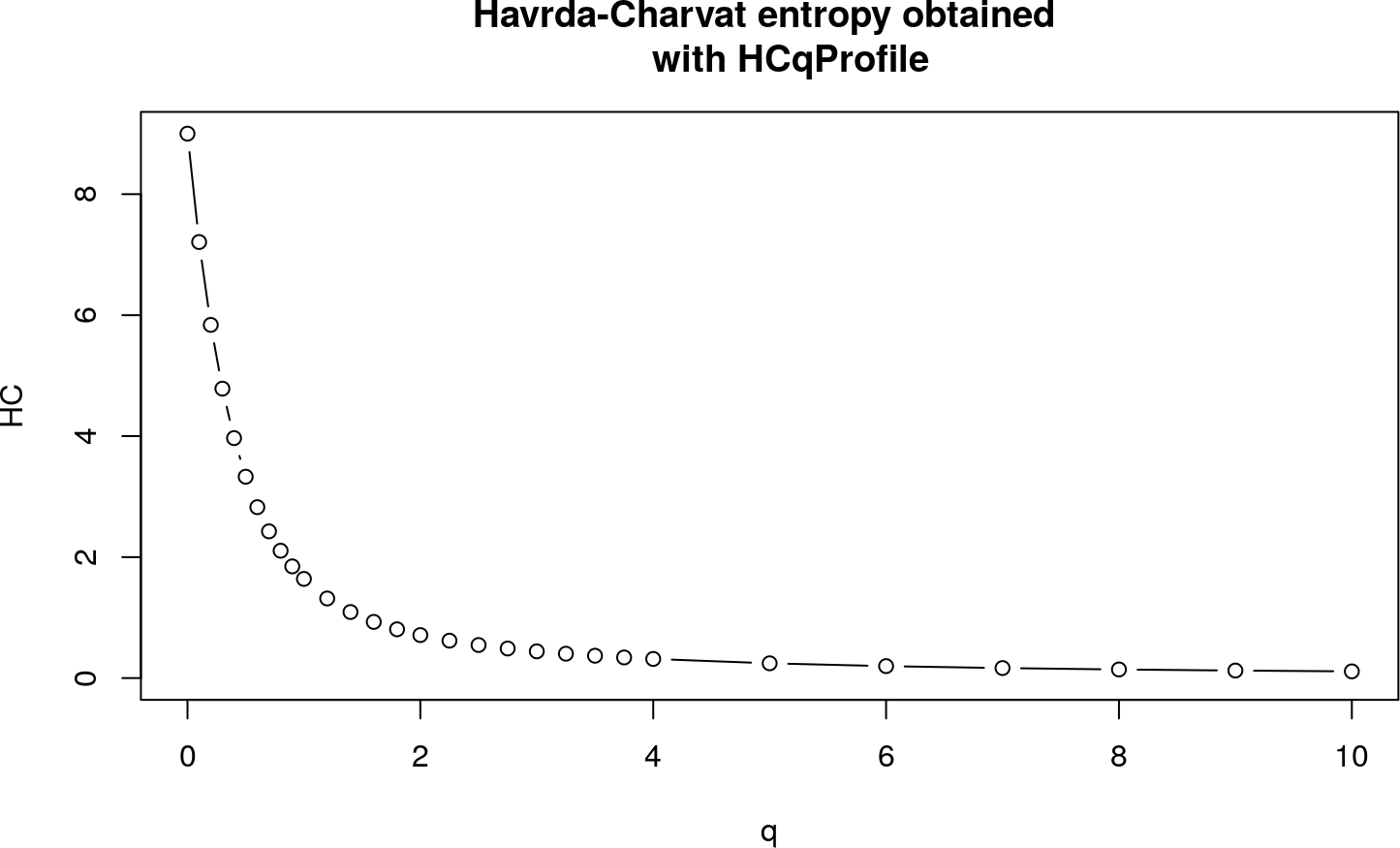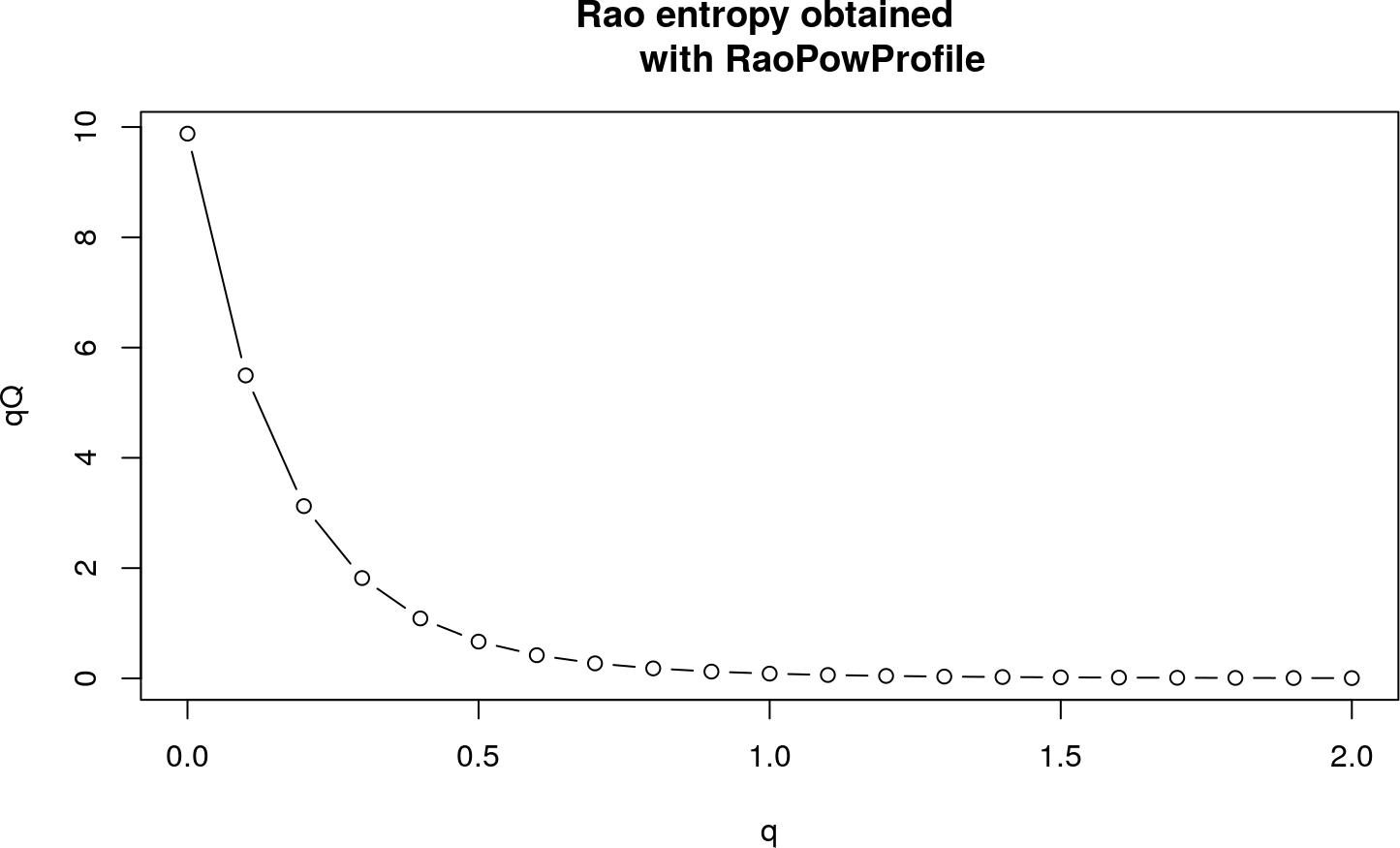# 1 Quasispecies complexity by biodiversity indices

RNA and DNA viruses that replicate by low fidelity polymerases generate a viral quasispecies, a collection of closely related viral genomes.

The high replication error rate that generates this quasispecies is due to a lack of proofreading mechanisms. This feature, in addition to the high replication rate of some viruses, leads to generation of many mutations in each cycle of viral replication.

The complexity of the quasispecies contributes greatly to the adaptive potential of the virus. The complexity of a viral quasispecies can be defined as an intrinsic property that quantifies the diversity and frequency of haplotypes, independently of the population size that contains them. (Gregori et al. 2016) (Gregori et al. 2014)

Quasispecies complexity can explain or predict viral behavior, so it has an obvious interest for clinical purposes. We are often interested in comparing the viral diversity indices between sequential samples from a single patient or between samples from groups of patients. These comparisons can provide information on the patient’s clinical progression or the appropriateness of a given treatment.

QSUtils is a package intended for use with quasispecies amplicon NGS data, but it could also be useful for analyzing 16S/18S ribosomal-based metagenomics or tumor genetic diversity by amplicons.

In this tutorial we illustrate the use of functions contained in the package to compute diversity indices, which can be classified into incidence-based, abundance-based, and functional indices.

First, the package should be installed and loaded.

BiocManager::install("QSutils")
library(QSutils)

Then, the dataset to work with the diversity indices should be loaded. This vignette deals with the different ways to load data: Simulation vignette for package users is also available.

filepath<-system.file("extdata","ToyData_10_50_1000.fna", package="QSutils")
lst
## $nr ##  464 62 39 27 37 16 33 54 248 20 ## ##$hseqs
##   A DNAStringSet instance of length 10
##      width seq                                           names
##      50 CACCCTGTGACCAGTGTGTGC...TGCCCCGTTGGCATTGACTAT Hpl_0_0001|464|46.4
##      50 CACTCTGTGACCAGTGTGTGC...TGCCCCGTTGGCATTGACTAT Hpl_1_0001|62|6.2
##      50 CACCCTGTGACCAGCGTGTGC...TGCCCCGTTGGCATTGACTAT Hpl_1_0002|39|3.9
##      50 CACCCTGTGACCAGTGTGTGC...TGCCCCGTTGGCATTGACTAC Hpl_1_0003|27|2.7
##      50 CACTCTGTGACCAGTGTGTGC...TGCCCCGTTAGCATTGACTAT Hpl_2_0001|37|3.7
##      50 CACTCGGTGACCAGTGTGCGT...TGCCCCGTTGGCATTGACTAT Hpl_4_0001|16|1.6
##      50 CACTCTGTGACCAGTGTGCGC...TGCCCTGTCGGCATTGACTAT Hpl_5_0001|33|3.3
##      50 CACTCTGTGATCAGTGTGCGC...TGCCCTGCCGGCATCGACTAT Hpl_8_0001|54|5.4
##      50 CACTCTGTGATCAGTGTGCGC...TGCCCTGCCGGCATCGACTAC Hpl_9_0001|248|24.8
##     50 CACTCTGTGATCAGTGTGCGC...TGCCCTGCCGGCACCGACTAC Hpl_10_0001|20|2

# 2 Incidence-based diversity indices or richness indices

Incidence-based diversity indices correspond to the number of observed entities, regardless of their abundances. The main incidence indices are the number of haplotypes, the number of polymorphic sites, and the number of mutations. These indices can be computed as follows:

• number of haplotypes

length(lst$hseqs) ##  10 In this fasta file, 10 haplotypes are reported. This can be calculated with the length of the DNAStringSet object. • The number of polymorphic sites SegSites(lst$hseqs)
##  14

In this example, there are 14 polymorphic sites; that is, mutated positions with respect to the dominant haplotype.

• Number of mutations

TotalMutations(lsthseqs) ##  37 Returns the total number of mutations observed in the alignment. # 3 Abundance-based diversity indices Abundance-based diversity indices take into account the entities present and their relative abundance in the population. • Shannon entropy Shannon(lstnr)
##  1.6396
NormShannon(lst$nr) ##  0.7120691 Shannon entropy is a measure of uncertainty. This index varies from 0, when there is a single haplotype, to the logarithm of the number of haplotypes. It represents the uncertainty in assigning a random genome to the corresponding haplotype in the population studied. This index is commonly used in the normalized form, although the non-normalized form is recommended. The normalized form constitutes a measure of evenness rather than diversity. The variance of Shannon entropy and normalized Shannon entropy can be computed with: ShannonVar(lst$nr)
##  0.001154642
NormShannonVar(lst$nr) ##  0.000217779 • Gini-Simpson GiniSimpson(lst$nr)
##  0.7117878
GiniSimpsonMVUE(lst$nr) ##  0.7117878 The Gini-Simpson index expresses the probability that two randomly sampled molecules from the viral population correspond to different haplotypes. It has a range of variation from 0, when there is a single haplotype, to 1 (asymptotically), when there are an infinity of equally abundant haplotypes. Interpretation of this index is clearer and more intuitive than that of Shannon entropy, and it is scarcely sensitive to rare haplotypes, as it gives higher weight to the more abundant variants. The variance of the Gini-Simpson index is obtained with: GiniSimpsonVar(lst$nr)
##  0.0128987
• Hill numbers

Although Shannon entropy, the Gini-Simpson index, and many other reported indices are considered measures of diversity, their units do not allow an easily interpreted and intuitive measure of true diversity. They are not linear with respect to the addition of new haplotypes, and they tend to saturate: the larger the number of haplotypes, the less sensitive they are to frequency changes. In contrast, Hill numbers are a generalization of diversity measures in units of equally abundant species.

Hill(lst$nr,q=1) ##  5.153107 Hill function computes the Hill number for a given order, q, but in QSutils there is a wrapper function that computes a profile of Hill numbers for different q values, so that one can see what happens when q increases in a plot. HillProfile(lst$nr)
plot(HillProfile(lst$nr),type ="b", main="Hill numbers obtained with HillProfile") ## q qD ## 1 0.00 10.000000 ## 2 0.10 9.364553 ## 3 0.20 8.751454 ## 4 0.30 8.166229 ## 5 0.40 7.613843 ## 6 0.50 7.098360 ## 7 0.60 6.622668 ## 8 0.70 6.188332 ## 9 0.80 5.795587 ## 10 0.90 5.443454 ## 11 1.00 5.153107 ## 12 1.20 4.607768 ## 13 1.40 4.203660 ## 14 1.60 3.891941 ## 15 1.80 3.650325 ## 16 2.00 3.461118 ## 17 2.25 3.278284 ## 18 2.50 3.138087 ## 19 2.75 3.028004 ## 20 3.00 2.939603 ## 21 3.25 2.867153 ## 22 3.50 2.806698 ## 23 3.75 2.755460 ## 24 4.00 2.711447 ## 25 5.00 2.583506 ## 26 6.00 2.501359 ## 27 7.00 2.444364 ## 28 8.00 2.402760 ## 29 9.00 2.371234 ## 30 10.00 2.346626 ## 31 Inf 2.155172Figure 1: Hill numbers profile As the order of the Hill number increases, the measure of diversity becomes less sensitive to rare haplotypes. Note that the Hill number of order q=0 is simply the number of haplotypes. For q=1 the Hill number is undefined, but as approaches 1 it tends to the exponential of Shannon entropy. For q=2 the Hill number corresponds to the inverse of the Simpson index and for q=Inf it is the inverse of the relative abundance of the rarest haplotype. • Rényi entropy Renyi(lst$nr,q=3)
##  1.078274

Rényi entropy is a log transformation of the Hill numbers.

RenyiProfile(lst$nr) plot(RenyiProfile(lst$nr),type ="b", main="Rényi entropy obtained with
RenyiProfile")
##        q     renyi
## 1   0.00 2.3025851
## 2   0.10 2.2369316
## 3   0.20 2.1692199
## 4   0.30 2.1000072
## 5   0.40 2.0299680
## 6   0.50 1.9598637
## 7   0.60 1.8904982
## 8   0.70 1.8226656
## 9   0.80 1.7570967
## 10  0.90 1.6944139
## 11  1.00 1.6395998
## 12  1.20 1.5277437
## 13  1.40 1.4359555
## 14  1.60 1.3589079
## 15  1.80 1.2948162
## 16  2.00 1.2415916
## 17  2.25 1.1873200
## 18  2.50 1.1436134
## 19  2.75 1.1079036
## 20  3.00 1.0782744
## 21  3.25 1.0533194
## 22  3.50 1.0320087
## 23  3.75 1.0135843
## 24  4.00 0.9974824
## 25  5.00 0.9491472
## 26  6.00 0.9168340
## 27  7.00 0.8937851
## 28  8.00 0.8766183
## 29  9.00 0.8634104
## 30 10.00 0.8529785
## 31   Inf 0.7678707Figure 2: Rényi entropy profile

RenyProfile, a wrapper function of Renyi, computes the Rényi entropy for a set of predefined q to compute a plot to see the behavior of this index as q increases.

• Havrda-Charvat estimator

HCq(lst$nr, q= 4) ##  0.3166118 Havrda-Charvat entropy, a measure of the diversity of each population, is a generalization of Shannon entropy. HCqVar(lst$nr, q= 4)
##  3.860805e-06

The asymptotic variance for a given exponent of this estimator can be computed with HCqVar.

HCqProfile(lst$nr) plot(HCqProfile(lst$nr),type ="b", main="Havrda-Charvat entropy obtained
with HCqProfile")
##        q        HC
## 1   0.00 9.0000000
## 2   0.10 7.2083625
## 3   0.20 5.8388239
## 4   0.30 4.7846529
## 5   0.40 3.9672588
## 6   0.50 3.3285494
## 7   0.60 2.8254117
## 8   0.70 2.4257164
## 9   0.80 2.1054156
## 10  0.90 1.8464291
## 11  1.00 1.6395998
## 12  1.20 1.3164050
## 13  1.40 1.0923685
## 14  1.60 0.9291887
## 15  1.80 0.8063456
## 16  2.00 0.7110760
## 17  2.25 0.6186440
## 18  2.50 0.5467412
## 19  2.75 0.4892160
## 20  3.00 0.4421381
## 21  3.25 0.4028962
## 22  3.50 0.3696911
## 23  3.75 0.3412423
## 24  4.00 0.3166118
## 25  5.00 0.2443882
## 26  6.00 0.1979576
## 27  7.00 0.1658853
## 28  8.00 0.1425482
## 29  9.00 0.1248749
## 30 10.00 0.1110596
## 31   Inf 0.0000000Figure 3: Havrda-Charvat entropy profile

The HCq function computes Havrda-Charvat entropy for a given order, q, and HCqProfile is a wrapper function that computes a profile of Havrda-Charvat entropy for different q values.

# 4 Functional diversity

Going a step further, functional diversity takes into account the differences between haplotypes in the quasispecies by considering their genetic distances.

## 4.1 Incidence-based functional diversity indices

This set of functions only considers the distances between haplotypes.

dst <- DNA.dist(lsthseqs,model="raw") library(psych) D <- as.matrix(dst) rownames(D) <- sapply(rownames(D),function(str) strsplit(str, split="\\|")[]) colnames(D) <- rownames(D) D ## Hpl_0_0001 Hpl_1_0001 Hpl_1_0002 Hpl_1_0003 Hpl_2_0001 ## Hpl_0_0001 0.00 0.02 0.02 0.02 0.04 ## Hpl_1_0001 0.02 0.00 0.04 0.04 0.02 ## Hpl_1_0002 0.02 0.04 0.00 0.04 0.06 ## Hpl_1_0003 0.02 0.04 0.04 0.00 0.06 ## Hpl_2_0001 0.04 0.02 0.06 0.06 0.00 ## Hpl_4_0001 0.08 0.06 0.10 0.10 0.08 ## Hpl_5_0001 0.10 0.08 0.12 0.12 0.10 ## Hpl_8_0001 0.16 0.14 0.18 0.18 0.16 ## Hpl_9_0001 0.18 0.16 0.20 0.16 0.18 ## Hpl_10_0001 0.20 0.18 0.22 0.18 0.20 ## Hpl_4_0001 Hpl_5_0001 Hpl_8_0001 Hpl_9_0001 Hpl_10_0001 ## Hpl_0_0001 0.08 0.10 0.16 0.18 0.20 ## Hpl_1_0001 0.06 0.08 0.14 0.16 0.18 ## Hpl_1_0002 0.10 0.12 0.18 0.20 0.22 ## Hpl_1_0003 0.10 0.12 0.18 0.16 0.18 ## Hpl_2_0001 0.08 0.10 0.16 0.18 0.20 ## Hpl_4_0001 0.00 0.10 0.16 0.18 0.20 ## Hpl_5_0001 0.10 0.00 0.06 0.08 0.10 ## Hpl_8_0001 0.16 0.06 0.00 0.02 0.04 ## Hpl_9_0001 0.18 0.08 0.02 0.00 0.02 ## Hpl_10_0001 0.20 0.10 0.04 0.02 0.00 • Average mutation frequency by entity MutationFreq(dst)  ##  0.082 The mean mutation frequency by entity, Mfe, measures the fraction of nucleotides in the haplotype alignment that differ from the dominant haplotype in the quasispecies • FAD FAD(dst) ##  9.88 The functional attribute diversity, FAD, is the sum of pairwise distances between haplotypes in the alignment. • Nucleotide diversity by entity NucleotideDiversity(dst)  ## [,1] ## [1,] 0.1097778 The nucleotide diversity by entity, $$\pi_e$$, is a transformation of FAD and it expresses the average difference between haplotypes in the alignment. ## 4.2 Abundance-based functional diversity indices • Average mutation frequency by molecule nm <- nmismatch(pairwiseAlignment(lsthseqs,lst$hseqs)) MutationFreq(nm=nm,nr=lst$nr,len=width(lst$hseqs)) ##  0.0659 The proportion of different nucleotides at the molecular level, Mfm, measures the fraction of nucleotides in the population that differ from the dominant haplotype in the quasispecies. MutationFreqVar(nm,lst$nr,len=width(lst$hseqs)) ##  6.41759e-06 The variance of Mfm is calculated with the MutationFreqVar function. • Nucleotide diversity NucleotideDiversity(dst,lst$nr)
##            [,1]
## [1,] 0.08634655

The nucleotide diversity, $$\pi_m$$, which is related to Rao entropy in ecology, corresponds to the mean genetic distance between molecules in the population.

• Rao entropy

RaoPow(dst,4,lst$nr) ##  6.332901e-05 To obtain the Rao entropy for a given order of q, the RaoPow function is used. RaoVar(dst,lst$nr)
##              [,1]
## [1,] 2.345394e-06

The variance of $$\pi_m$$ is calculated with the RaoVar function.

RaoPowProfile(dst,lst$nr) plot(RaoPowProfile(dst,lst$nr),type ="b", main="Rao entropy obtained
with RaoPowProfile")
##      q          qQ
## 1  0.0 9.880000000
## 2  0.1 5.494606337
## 3  0.2 3.124207222
## 4  0.3 1.819585453
## 5  0.4 1.087184512
## 6  0.5 0.667121028
## 7  0.6 0.420633660
## 8  0.7 0.272493135
## 9  0.8 0.181233347
## 10 0.9 0.123589240
## 11 1.0 0.086260200
## 12 1.1 0.061491451
## 13 1.2 0.044668953
## 14 1.3 0.032989839
## 15 1.4 0.024715352
## 16 1.5 0.018743915
## 17 1.6 0.014362892
## 18 1.7 0.011101667
## 19 1.8 0.008643155
## 20 1.9 0.006769532
## 21 2.0 0.005328380Figure 4: Rao entropy profile

# 5 Sample size and bias

The diversity we measure in viral quasispecies samples is necessarily tied to the sample size. The larger the coverage, the larger the number of haplotypes, polymorphic sites, and mutations we can find. The same is true for Shannon entropy and other diversity indices. Because of the variability in all experimental procedures, the best pooling of samples never results in perfectly balanced coverage, which complicates the comparison of diversity indices biased by sample size. Hence, a sample size correction method is required to obtain fair inferences. The literature regarding ecology shows several correction methods, which unfortunately, are not applicable to NGS because of one differential trait. With NGS, sooner or later we are obliged to eliminate all haplotypes present in frequencies below a threshold, considered the noise level of the technique. This lower end of frequencies contains the information required by most established corrections.

The correction we found that works best in this situation consists in determining the minimum coverage of all samples to be compared and down sampling the other samples to this minimum, simply by rescaling. All haplotypes with resulting frequencies below a given threshold are then removed with high confidence, in a step we have dubbed fringe trimming. The $$DSFT$$ function is used to correct the quasispecies composition to a given sample size. It takes four parameters: $$nr$$, the vector of haplotype frequencies in the original sample; $$size$$, the total number of reads in the new sample prior to abundance filtering; $$p.cut$$, the abundance threshold in the filter; and $$conf$$, the level of confidence in trimming haplotypes, which defaults to 0.95. $$DSFT$$ returns a vector of logicals with FALSE for all haplotypes to be removed by the correction.

The next code shows the impact of each data treatment step when determining Shannon entropy. First, we simulate the frequencies of haplotypes in a quasispecies population. Then we take repeated samples from this population, 2000 and 5000 reads in size. We then compute Shannon entropy of the raw samples,and the samples after noise filtering at an abundance of 0.1%, and after the DSFT corrections at size 2000, and filtering at 0.2% with 95% confidence.

set.seed(123)
n <- 2000
y <- geom.series(n,0.8)+geom.series(n,0.00025)
nr.pop <- round(y*1e7)

thr <- 0.1
sz1 <- 5000
sz2 <- 10000

qs.sample <- function(nr.pop,sz1,sz2){
div <- numeric(6)
nr.sz1 <- table(sample(length(nr.pop),size=sz1,replace=TRUE,p=nr.pop))
div <- Shannon(nr.sz1)
nr.sz1 <- nr.sz1[nr.sz1>=sz1*thr/100]
div <- Shannon(nr.sz1)
div <- Shannon(nr.sz1[DSFT(nr.sz1,sz1)])

nr.sz2 <- table(sample(length(nr.pop),size=sz2,replace=TRUE,p=nr.pop))
div <- Shannon(nr.sz2)
nr.sz2 <- nr.sz2[nr.sz2>=sz2*thr/100]
div <- Shannon(nr.sz2)
div <- Shannon(nr.sz2[DSFT(nr.sz2,sz1)])
div
}

hpl.sim <- replicate(2000,qs.sample(nr.pop,sz1,sz2))
nms <- paste(c(sz1,sz2))

par(mfrow=c(1,3))

boxplot(t(hpl.sim[1:2,]),names=nms,col="lavender",las=2,
ylab="Shannon entropy",main="raw")
boxplot(t(hpl.sim[3:4,]),names=nms,col="lavender",las=2,
ylab="Shannon entropy",main="filt")
boxplot(t(hpl.sim[5:6,]),names=nms,col="lavender",las=2,
ylab="Shannon entropy",main="DSFT")